Published: 05 February 2022

# Dynamical characteristics and vibration behavior of the novel composite dry separator

Hongxi Li1
Enhui Zhou2
Ling Shen3
Zixin Yin4
Zhang Bo5
1School of Mechanical Engineering, China University of Mining and Technology, Xuzhou Jiangsu, 221116, China
2, 5School of Chemical, China University of Mining and Technology, Xuzhou Jiangsu, 221116, China
3School of Physics, China University of Mining and Technology, Xuzhou Jiangsu, 221116, China
4School of Mechanical and Electronic Engineering, Suzhou University, Suzhou Anhui, 234000, China
Corresponding Author:
Enhui Zhou
Views 55

#### Abstract

At present, the composite dry separation technology is a successful technology in coal gangue discharge. In this paper, the mechanical structure of the novel composite dry separator (NCDS) was revealed, and the dynamic characteristics and vibration behaviors of the separator were deeply discussed by combining theoretical modeling and experimental testing methods. The dynamic mechanics mathematical model of the NCDS was established. The dynamic experiment of the separator was carried out by using the vibration testing system to reveal the space-time characteristics of the NCDS and compared with the theoretical results. The results show that the error between experimental data and theoretical data is less than 6.78 %. This work should be useful for optimal design and efficient operation of the composite dry separator.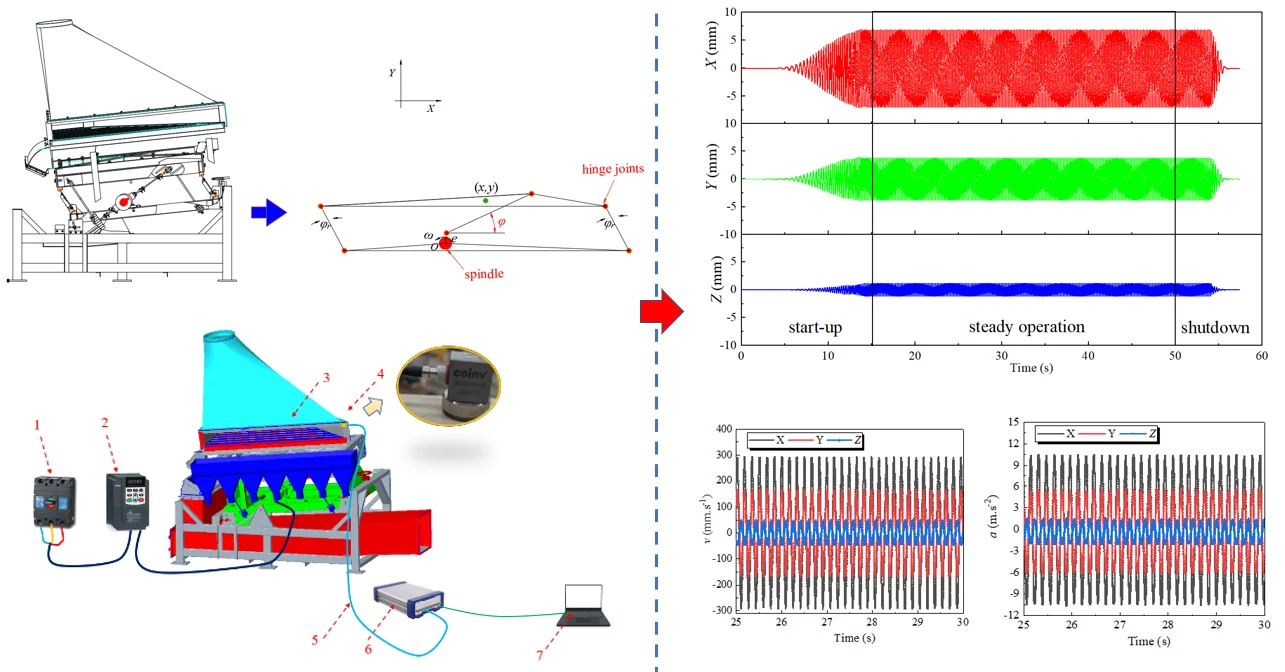#### Highlights

• The kinematic model of a Novel Composite Dry Separator was proposed
• The vibration of the separator was examined by theoretical calculation and experimental test
• The dynamic characteristics of the separator were revealed

## 1. Introduction

China is the world’s largest producer and consumer of coal [1, 2]. Coal preparation is the basis and premise of realizing clean coal utilization, energy saving and sustainable development, and it is also the most economical and effective method of clean coal technology [3, 4]. For the coal resources with abundant reserves in northwest of China where is extremely short of water, dry coal preparation technology is urgently needed. Dry coal separation has more advantages than wet coal separation [5-7].

The composite dry separator is mainly used for discharging gangue of steam coal and coking coal [8, 9]. The composite dry separator uses the powder contained in the feed as self-generating medium to form gas-solid mixture. Material mainly subjected to the comprehensive effect of vibration and wind to complete the stratification and realize the separation process according to density. Research on the design theory and equipment of the composite dry separator is of great significance to improve the raw coal selection rate, reduce the cost of coal washing and realize the efficient and clean utilization of coal by providing technical support and equipment support for large coal preparation plants [10, 11].

## 2. Modeling

### 2.1. Mechanical structure of the novel composite dry separator

The mechanical structure of the novel composite dry separator (NCDS) which used in Shandong China is shown in Fig. 1. The NCDS is composed of air supply system and main machine system. The main machine system includes the separation bed, the supporting structure, the feeding and discharging ports and the driving system. The separation bed is connected with the supporting structure by bolts, plate spring and eccentric sleeve and bearing.

The spindle is fixed on the supporting structure through the bearing seats, and driven by the frequency conversion motor through the pulley and belt; The motor adjusts the output rotation speed through the frequency converter; The spindle drives the eccentric sleeve, and the eccentric sleeve drives the separation bed reciprocating movement through the plate spring. The vibration intensity is closely related to the performance of the NCDS. Therefore, it plays a key role in fully understanding the working performance of the NCDS to reveal the dynamic characteristics and the vibration behavior.

Fig. 1Mechanical structure of the novel composite dry separator (NCDS)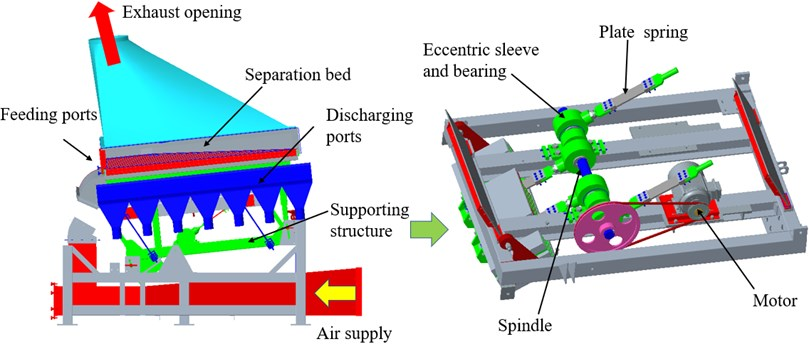### 2.2. Dynamical model of the novel composite dry separator

The dynamical model of the NCDS is shown in Fig. 2. The spindle and hinge joints were painted red. During the working process, the spindle moves in a uniform circular motion, and the separation bed moves in a reciprocating motion which similar to a straight line. The displacement, velocity and acceleration of the separation bed are constantly changing. Therefore, it is of great significance to study the motion characteristics of the separation bed. In order to study the motion characteristics of separation bed, the absolute coordinate system $OXY$ was established with the rotating center of the spindle as the origin $O$, the $X$ axis was parallel to the separation bed surface and pointed to the direction of the material flow, and the $Y$ axis was perpendicular to the separation bed surface. According to the working principle, the NCDS was simplified and treated equivalent. $e$ is the eccentricity, $\omega$ is the spindle rotational speed.

Fig. 2Dynamical model of the NCDS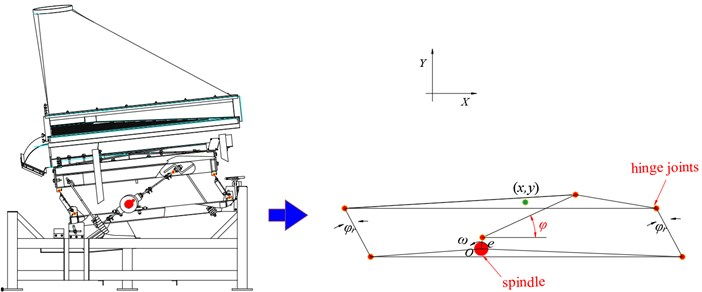When the spindle rotates, the displacement of the separation bed can be expressed as:

1
$\left\{\begin{array}{c}x=ecos\phi cos\omega t,\\ y=esin\phi cos\omega t,\end{array}\right\$

where, ($x$, $y$) are the displacements of the separation bed along the $X$ and $Y$ directions, $\phi$ is the inclination angle between the plate spring and the separation bed. The first derivative and second derivative of Eq. (1) with respect to time t can be obtained respectively, and the velocity and acceleration of the selected bed can be scored, as shown in Eq. (2):

2
$\left\{\begin{array}{c}\stackrel{˙}{x}=-e\omega cos\phi sin\omega t,\\ \stackrel{¨}{x}=-e{\omega }^{2}cos\phi cos\omega t,\\ \stackrel{˙}{y}=-e\omega sin\phi sin\omega t,\\ \stackrel{¨}{y}=-e{\omega }^{2}sin\phi cos\omega t,\end{array}\right\$

The displacement amplitude of the separation bed (${A}_{x},{A}_{y}$) can be expressed as Eq. (3):

3
$\left\{\begin{array}{c}{A}_{x}=ecos\phi ,\\ {A}_{y}=esin\phi .\end{array}\right\$

## 3. Experiment and analysis

### 3.1. Experiment preparation

The experiment and analysis system for dynamic characteristics of the NCDS is shown in Fig. 3. The system mainly includes: 1 circuit breaker, 2 frequency converter, 3 NCDS, 4 ICP three direction acceleration sensor, 5 Cable, 6 INV3060S multi-channel signal acquisition instrument, 7 Coinv DASP multi-channel signal real-time analysis software.

Fig. 3Vibration experiment and analysis system of the NCDS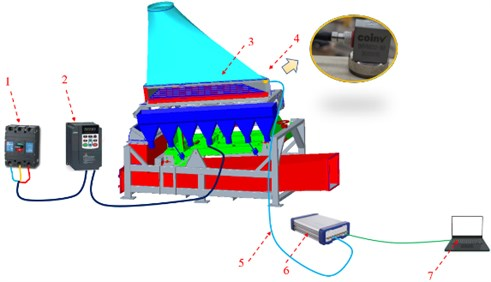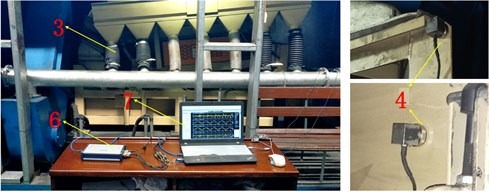The acceleration sensors were fixed on the separation bed of the NCDS by magnetic seat. The $X$-axis direction of the acceleration sensor was parallel to the separation bed, the $Y$-axis direction was perpendicular to the separation bed, and the $Z$-axis direction was perpendicular to the side plate. The acceleration sensors were connected to the multi-channel acquisition instrument through a cable. The multi-channel acquisition instrument was connected to a computer which installed Coinv DASP software. A circuit breaker was used to control the opening and closing of the power. Adjust the frequency converter to set the rotational speed of the driving motor. Before each test, check the circuit first, then turn on the circuit breaker to switch on the power supply, and then turn on the Coinv DASP software for data collection. Turn on the running button of the frequency converter, start the motor, record about 60 seconds, then turn off the frequency converter. When the vibration of the NCDS completely stopped, shut down the Coinv DASP software signal collection and save the data. When all tests are completed, turn off the circuit breaker.

### 3.2. Analysis of time-frequency response

The dynamic parameters of the NCDS were analyzed after the vibration experiment. Fig. 4 shows the displacement time-domain response curves of the separation bed of the NCDS with the spindle rotational speed $n$ of 370 rpm ($\omega =$38.73 rad/s). The time-domain response curves can be divided into three different stages: start-up stage (0-20 s), steady operation stage (20-50 s) and shutdown stage (50-60 s). The displacement signals in each stage are obviously different. In the start-up stage (0-20 s), the spindle rotational speed increased gradually from 0 to 370 rpm driven by the driving motor. In this process, the vibration frequency of the NCDS was gradually accelerated and the amplitude of the displacement was increased simultaneously. In the steady operation stage (20-50 s), the driving motor reached the working rotational speed, the displacement amplitude of the NCDS was stable, and the vibration frequency was consistent with the driving motor; During the steady operation stage, the displacement amplitude of the NCDS was 6.88 mm in $X$ direction and 3.78 mm in $Y$ direction. The displacement amplitude in the $Z$ direction was relatively small which can be ignored. During the shutdown stage (50-60 s), the displacement amplitude of the NCDS was gradually decreasing and the vibration frequency was getting slower, and finally the NCDS stopped.

Fig. 4Time-responses of displacement about the NCDS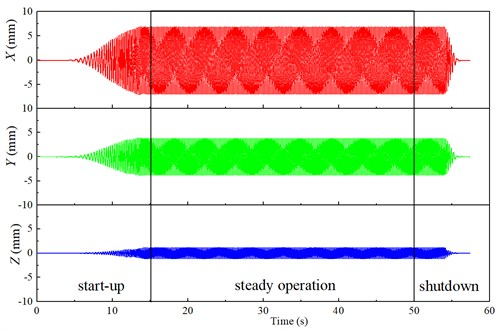According to the time-domain response results, the displacement spatial and temporal characteristic curves in different periods can be obtained, as shown in Fig. 5. The spatial and temporal characteristic curve can directly reflect the space movement trajectory of the NCDS. In start-up stage (0-20 s) and shutdown stage (50-60 s), the spatial and temporal characteristic curves of displacement were complicated. In steady operation stage (20-50 s), the spatial and temporal characteristic curve of displacement was a standard oblique line. The inclination angle of oblique line was $\phi$ which was the inclination angle between the plate spring and the separation bed.

Fig. 5Spatial and temporal characteristic curve of displacement about the NCDS in different time periods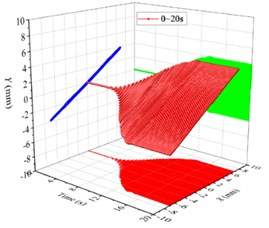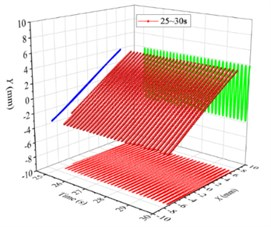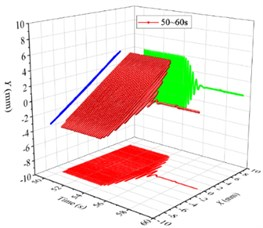Fig. 6Time-responses of velocity and acceleration about the NCDS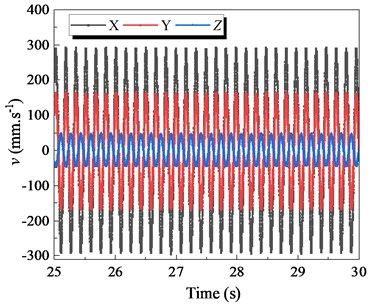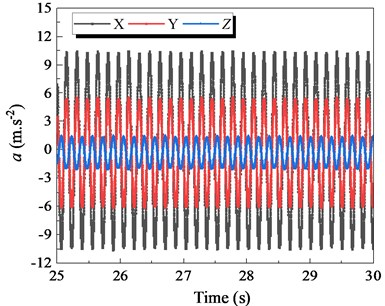In addition, due to the high vibration frequency of the NCDS, the velocity and acceleration are considered to be important indicators of vibration intensity. Fig. 6 shows the time-domain variation curves of the velocity and acceleration of the NCDS along the $X$, $Y$ and $Z$ axis in the steady operation stage. Similar to the time-domain response curve of displacement, the velocity direction of the NCDS was always the same along the $X$ and $Y$ axis with the phase difference of 0°. The $X$-axis velocity amplitude of the NCDS was 287.60 mm∙s-1, and the $Y$-direction velocity amplitude was 161.6 mm∙s-1. The $X$-axis acceleration amplitude of the separator was 10.43 m∙s-2, and the $Y$-direction acceleration amplitude was 6.25 m∙s-2. The velocity and acceleration amplitude of $X$ axis are obviously larger than that of $Y$ direction. In addition, the amplitudes of velocity and acceleration in $Y$ direction were smaller than that in $X$ direction, which was beneficial to keep the overall vibration characteristics unchanged and reduce the vibration of the NCDS itself in the process of separation. The velocity and acceleration of the NCDS along the $Z$ axis are relatively small, and the phases along the $Z$ axis differ from those along the $X$ and $Y$ axes by 180°.

Table 1Comparisons of theoretical results and experimental results

 Parameter Theoretical results Experimental results Error (%) $\left|x\right|$ (mm) 6.93 6.88 0.73 $\left|\stackrel{˙}{x}\right|$ (mm∙s-1) 268.1 287.6 –6.78 $\left|\stackrel{¨}{x}\right|$ (m∙s-2) 10.4 10.43 –0.29 $\left|y\right|$ (mm) 4.0 3.78 5.56 $\left|\stackrel{˙}{y}\right|$ (mm∙s-1) 154.7 161.6 –4.27 $\left|\stackrel{¨}{y}\right|$ (m∙s-2) 5.98 5.92 1.00

The comparison between the theoretical calculation results of the displacement amplitude, velocity amplitude and acceleration amplitude of the NCDS based on the dynamic model and the experimental results is shown in Table 1. It can be seen from the table that the theoretical calculation results are in good agreement with the experimental results. The maximum relative error is only 6.78 %. It shows that the theoretical calculation results have high reliability and accuracy.

## 4. Conclusions

1) The mechanical structure principle of the novel composite dry separator (NCDS) was revealed. The dynamics theoretical model of the NCDS was deduced, and the dynamical characteristics were investigated by the theoretical and vibration experiment analysis.

2) The time-domain response curves of displacement, velocity and acceleration, and spatial trajectory were obtained. The trajectory of the NCDS was a standard oblique line. The directions of displacement, velocity and acceleration of the NCDS were always the same along the $X$ and $Y$ axis. The vibrations along the $Z$ axis were relatively small and the phases along the $Z$ axis differ from those along the $X$ and $Y$ axes by 180°.

3) The theoretical model was high reliable and accurate with maximum error of 6.78 %. This study provides theoretical and technical support for research and industrial applications of NCDS.

#### Cited by

Dynamics Analysis and Experiment of Novel Compound Dry Separator

21 December 2021
Accepted
14 January 2022
Published
05 February 2022
SUBJECTS
Mechanical vibrations and applications
Keywords
NCDS
dynamic characteristics
vibration experiment
space-time curve
Acknowledgements

This work is financially supported by the National Natural Science Foundation of China (51904298), the Doctoral Scientific Research Foundation of Suzhou University (2020BS004).